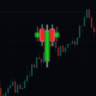# Fibonacci Breakout Target Levels

DiupdateFibonacci Extension

Fibonacci Extension is a powerful technical analysis tool that traders use to predict where the market might find support and resistance . It is based on the Fibonacci sequence and uses levels that are found by extending the 23.6%, 38.2%, 50%, 61.8%, and 100% Fibonacci ratios from a swing high or low. These levels can be used to find possible areas of support and resistance , and traders often use them to figure out when to get into or get out of a trade.

What does this indicator do?

This indicator gets five levels of the Fibonacci Extension and uses it for both the low and the high. The default lookback period is 10 days, and it checks for the highest and lowest price in that period. Then it calculates the extension levels and plots them, and it also adds a line that shows you the current breakout target levels.

How to use?

The primary use intended for this indicator is to be used to determine possible breakout target levels. Let's say you are trading a range and a breakout happens. You can use this indicator to determine possible take-profit zones and possible support and resistance zones.

Features:
• Change the lookback period for the Fibonacci Extension levels.
• Disable the Fibonacci Bands if you just want to see the FIB levels.
• You can also change the 5 levels and add different Fibonacci numbers.

In this image, you can see how you can use this indicator to determine take-profit levels. The Fibonacci Extensions will determine potential support and resistance levels, which could be good places to exit your long or short positions.

Catatan Rilis:
Just changed some of the comments in the script..